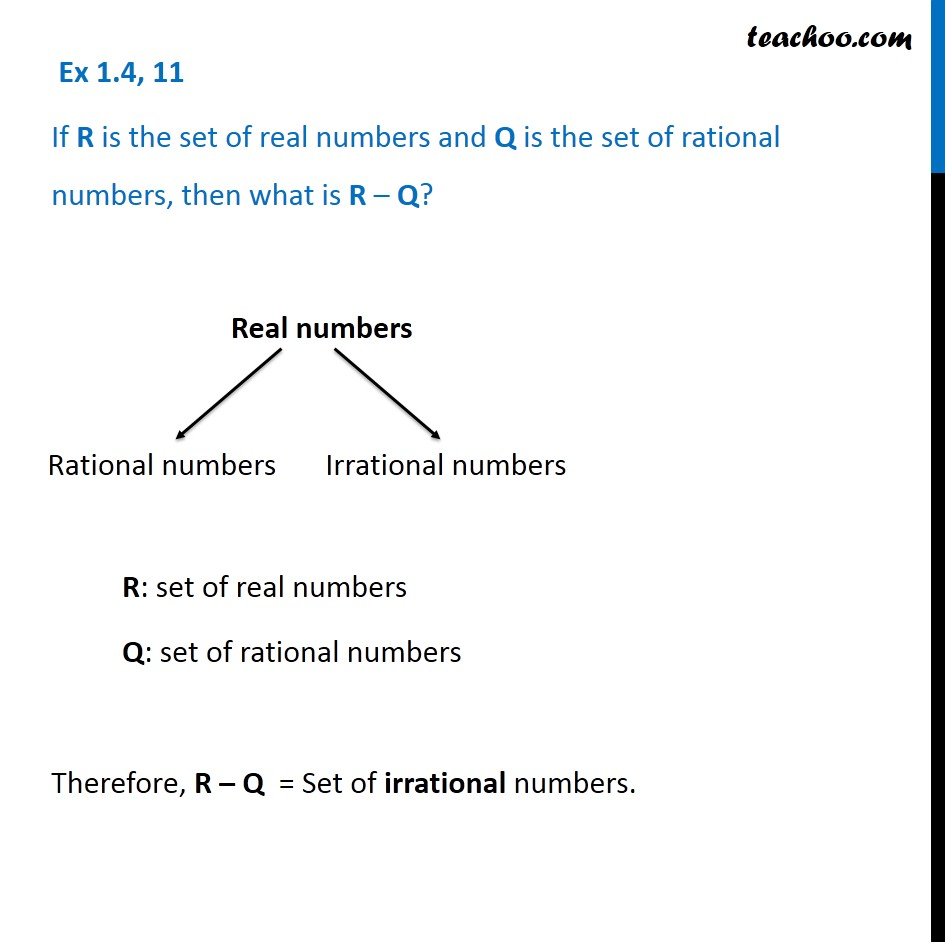1. Chapter 1 Class 11 Sets (Term 1)
2. Concept wise
3. Difference of sets

Transcript

Ex 1.4, 11 If R is the set of real numbers and Q is the set of rational numbers, then what is R – Q? R: set of real numbers Q: set of rational numbers Therefore, R – Q = Set of irrational numbers. Rational numbers Irrational numbers